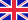•# Poincare' Series Associated to Milnor Algebras

Suppose that f is a homogeneous polynomial with complex coefficients. Let M(f) denotes the corresponding Milnor algebra, and V(f) the hypersurface defined by the equation f=0 in the complex projective space. The algebra M(f) is a graded C-algebra, where... Viac o knihe

Na objednávku, dodanie 2-4 týždne

44.35 €

bežná cena: 50.40 €

## O knihe

Suppose that f is a homogeneous polynomial with complex coefficients. Let M(f) denotes the corresponding Milnor algebra, and V(f) the hypersurface defined by the equation f=0 in the complex projective space. The algebra M(f) is a graded C-algebra, where C is the set of complex numbers. The aim of this Thesis is to determine the Poincar'e series of the Milnor algebra M(f) in terms of the geometry of the hypersurface V(f). The result is classically known for the case when V(f) is smooth. The goal of this research is to discuss the case when V(f) has only isolated singularities.

• Vydavateľstvo: LAP LAMBERT Academic Publishing
• Rok vydania: 2010
• Formát: Paperback
• Rozmer: 220 x 150 mm
• Jazyk: Anglický jazyk
• ISBN: 9783838395746

Generuje redakčný systém BUXUS CMS spoločnosti ui42.